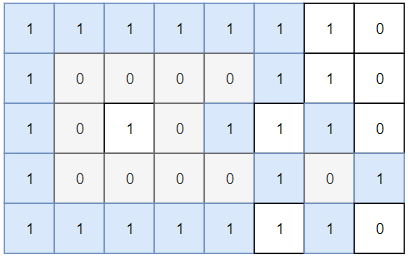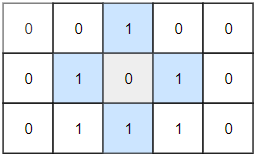1254. 统计封闭岛屿的数目

# 1254. 统计封闭岛屿的数目#

• 标签：深度优先搜索、广度优先搜索、并查集、数组、矩阵
• 难度：中等

## 题目大意 #

• $1 \le grid.length, grid.length \le 100$。
• $0 \le grid[i][j] \le 1$。

• 示例 1：1 2 3  输入：grid = [[1,1,1,1,1,1,1,0],[1,0,0,0,0,1,1,0],[1,0,1,0,1,1,1,0],[1,0,0,0,0,1,0,1],[1,1,1,1,1,1,1,0]] 输出：2 解释：灰色区域的岛屿是封闭岛屿，因为这座岛屿完全被水域包围（即被 1 区域包围）。 
• 示例 2：1 2  输入：grid = [[0,0,1,0,0],[0,1,0,1,0],[0,1,1,1,0]] 输出：1 

## 解题思路 #

### 思路 1：深度优先搜索 #

1. grid[i][j] == 0 的位置出发，使用深度优先搜索的方法遍历上下左右四个方向上相邻区域情况。
1. 如果上下左右都是 grid[i][j] == 1，则返回 True
2. 如果有一个以上方向的 grid[i][j] == 0，则返回 False
3. 遍历之后将当前陆地位置置为 1，表示该位置已经遍历过了。
2. 最后统计出上下左右都满足 grid[i][j] == 1 的情况数量，即为答案。

### 思路 1：代码 #

  1 2 3 4 5 6 7 8 9 10 11 12 13 14 15 16 17 18 19 20 21 22 23 24 25 26 27  class Solution: directs = [(0, 1), (0, -1), (1, 0), (-1, 0)] def dfs(self, grid, i, j): n, m = len(grid), len(grid) if i < 0 or i >= n or j < 0 or j >= m: return False if grid[i][j] == 1: return True grid[i][j] = 1 res = True for direct in self.directs: new_i = i + direct new_j = j + direct if not self.dfs(grid, new_i, new_j): res = False return res def closedIsland(self, grid: List[List[int]]) -> int: res = 0 for i in range(len(grid)): for j in range(len(grid)): if grid[i][j] == 0 and self.dfs(grid, i, j): res += 1 return res 

### 思路 1：复杂度分析 #

• 时间复杂度：$O(m \times n)$。其中 $m$ 和 $n$ 分别为行数和列数。
• 空间复杂度：$O(m \times n)$。